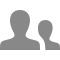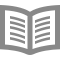## このコースについて

18,621 最近の表示

#### 柔軟性のある期限

スケジュールに従って期限をリセットします。

#### 柔軟性のある期限

スケジュールに従って期限をリセットします。

### Gregory Plettトップ講師陣

Professor
Electrical and Computer Engineering受講者8,1695 コース

### 提供：1

5時間で修了

## The importance of a good SOC estimator

5時間で修了
8件のビデオ （合計120分）, 13 readings, 7 quizzes
8件のビデオ
3.1.2: What is the importance of a good SOC estimator?8 分
3.1.3: How do we define SOC carefully?16 分
3.1.4: What are some approaches to estimating battery cell SOC?26 分
3.1.5: Understanding uncertainty via mean and covariance17 分
3.1.6: Understanding joint uncertainty of two unknown quantities15 分
3.1.7: Understanding time-varying uncertain quantities22 分
3.1.8: Summary of "The importance of a good SOC estimator" and next steps3 分
13件の学習用教材
Notes for lesson 3.1.11 分
Course Resources5 分
How to Use Discussion Forums5 分
Earn a Course Certificate5 分
Notes for lesson 3.1.21 分
Notes for lesson 3.1.31 分
Notes for lesson 3.1.41 分
Introducing a new element to the course!10 分
Notes for lesson 3.1.51 分
Notes for lesson 3.1.61 分
Notes for lesson 3.1.71 分
Notes for lesson 3.1.81 分
7の練習問題
Practice quiz for lesson 3.1.210 分
Practice quiz for lesson 3.1.310 分
Practice quiz for lesson 3.1.410 分
Practice quiz for lesson 3.1.515 分
Practice quiz for lesson 3.1.610 分
Practice quiz for lesson 3.1.76 分
Quiz for week 140 分
2

3時間で修了

## Introducing the linear Kalman filter as a state estimator

3時間で修了
6件のビデオ （合計97分）, 6 readings, 6 quizzes
6件のビデオ
3.2.2: The Kalman-filter gain factor23 分
3.2.3: Summarizing the six steps of generic probabilistic inference9 分
3.2.4: Deriving the three Kalman-filter prediction steps21 分
3.2.5: Deriving the three Kalman-filter correction steps16 分
3.2.6: Summary of "Introducing the linear KF as a state estimator" and next steps2 分
6件の学習用教材
Notes for lesson 3.2.11 分
Notes for lesson 3.2.21 分
Notes for lesson 3.2.31 分
Notes for lesson 3.2.41 分
Notes for lesson 3.2.51 分
Notes for lesson 3.2.61 分
6の練習問題
Practice quiz for lesson 3.2.112 分
Practice quiz for lesson 3.2.210 分
Practice quiz for lesson 3.2.310 分
Practice quiz for lesson 3.2.410 分
Practice quiz for lesson 3.2.510 分
Quiz for week 230 分
3

4時間で修了

## Coming to understand the linear Kalman filter

4時間で修了
7件のビデオ （合計86分）, 7 readings, 7 quizzes
7件のビデオ
3.3.2: Introducing Octave code to generate correlated random numbers15 分
3.3.3: Introducing Octave code to implement KF for linearized cell model10 分
3.3.4: How do we improve numeric robustness of Kalman filter?10 分
3.3.5: Can we automatically detect bad measurements with a Kalman filter?14 分
3.3.6: How do I initialize and tune a Kalman filter?12 分
3.3.7: Summary of "Coming to understand the linear KF" and next steps2 分
7件の学習用教材
Notes for lesson 3.3.11 分
Notes for lesson 3.3.21 分
Notes for lesson 3.3.31 分
Notes for lesson 3.3.41 分
Notes for lesson 3.3.51 分
Notes for lesson 3.3.61 分
Notes for lesson 3.3.71 分
7の練習問題
Practice quiz for lesson 3.3.110 分
Practice quiz for lesson 3.3.210 分
Practice quiz for lesson 3.3.310 分
Practice quiz for lesson 3.3.410 分
Practice quiz for lesson 3.3.510 分
Practice quiz for lesson 3.3.610 分
Quiz for week 330 分
4

4時間で修了

## Cell SOC estimation using an extended Kalman filter

4時間で修了
8件のビデオ （合計101分）, 8 readings, 7 quizzes
8件のビデオ
3.4.2: Deriving the three extended-Kalman-filter prediction steps15 分
3.4.3: Deriving the three extended-Kalman-filter correction steps6 分
3.4.4: Introducing a simple EKF example, with Octave code15 分
3.4.5: Preparing to implement EKF on an ECM20 分
3.4.6: Introducing Octave code to initialize and control EKF for SOC estimation13 分
3.4.7: Introducing Octave code to update EKF for SOC estimation16 分
3.4.8: Summary of "Cell SOC estimation using an EKF" and next steps2 分
8件の学習用教材
Notes for lesson 3.4.11 分
Notes for lesson 3.4.21 分
Notes for lesson 3.4.31 分
Notes for lesson 3.4.41 分
Notes for lesson 3.4.51 分
Notes for lesson 3.4.61 分
Notes for lesson 3.4.71 分
Notes for lesson 3.4.81 分
7の練習問題
Practice quiz for lesson 3.4.110 分
Practice quiz for lesson 3.4.210 分
Practice quiz for lesson 3.4.310 分
Practice quiz for lesson 3.4.410 分
Practice quiz for lesson 3.4.510 分
Practice quiz for lesson 3.4.710 分
Quiz for week 430 分

## Algorithms for Battery Management Systems専門講座について

In this specialization, you will learn the major functions that must be performed by a battery management system, how lithium-ion battery cells work and how to model their behaviors mathematically, and how to write algorithms (computer methods) to estimate state-of-charge, state-of-health, remaining energy, and available power, and how to balance cells in a battery pack....## よくある質問

• 修了証に登録すると、すべてのビデオ、テスト、およびプログラミング課題（該当する場合）にアクセスできます。ピアレビュー課題は、セッションが開始してからのみ、提出およびレビューできます。購入せずにコースを検討することを選択する場合、特定の課題にアクセスすることはできません。